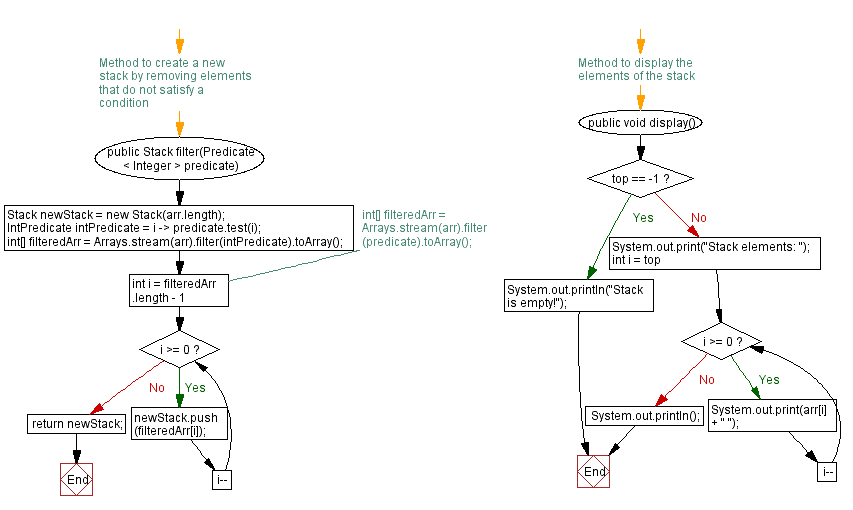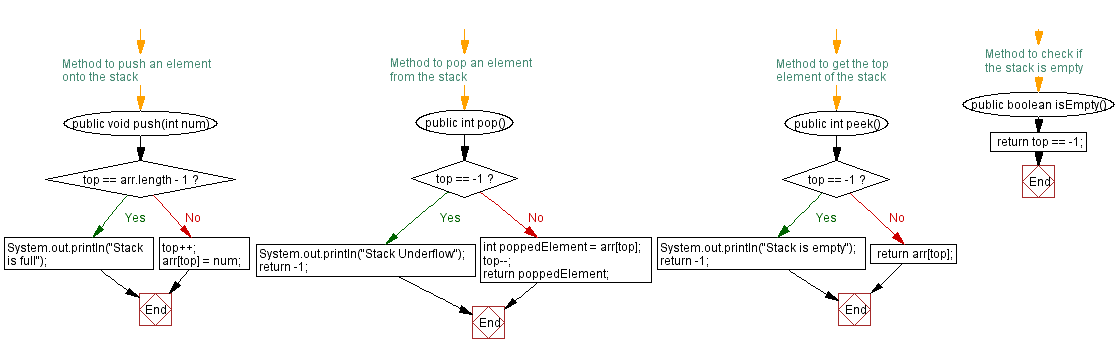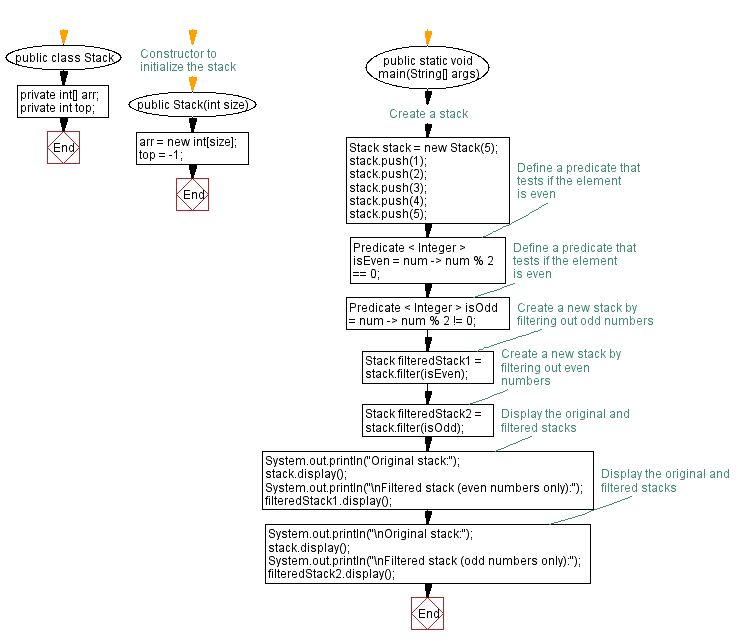# Java Exercises: Remove elements from a stack that do not meet a condition

## Java Stack: Exercise-28 with Solution

Write a Java program that implements a stack and create a new stack by removing elements that do not satisfy a condition.

Sample Solution:

Java Code:

``````import java.util.*;
import java.util.function.Predicate;
import java.util.Arrays;
import java.util.function.IntPredicate;

public class Stack {
private int[] arr;
private int top;

// Constructor to initialize the stack
public Stack(int size) {
arr = new int[size];
top = -1;
}

// Method to push an element onto the stack
public void push(int num) {
if (top == arr.length - 1) {
System.out.println("Stack is full");
} else {
top++;
arr[top] = num;
}
}

// Method to pop an element from the stack
public int pop() {
if (top == -1) {
System.out.println("Stack Underflow");
return -1;
} else {
int poppedElement = arr[top];
top--;
return poppedElement;
}
}

// Method to get the top element of the stack
public int peek() {
if (top == -1) {
System.out.println("Stack is empty");
return -1;
} else {
return arr[top];
}
}

// Method to check if the stack is empty
public boolean isEmpty() {
}

// Method to get the size of the stack
public int getSize() {
}

// Method to create a new stack by removing elements that do not satisfy a condition
public Stack filter(Predicate < Integer > predicate) {
Stack newStack = new Stack(arr.length);

IntPredicate intPredicate = i -> predicate.test(i);
int[] filteredArr = Arrays.stream(arr).filter(intPredicate).toArray();

//int[] filteredArr = Arrays.stream(arr).filter(predicate).toArray();
for (int i = filteredArr.length - 1; i >= 0; i--) {
newStack.push(filteredArr[i]);
}
return newStack;
}

// Method to display the elements of the stack
public void display() {
if (top == -1) {
System.out.println("Stack is empty!");
} else {
System.out.print("Stack elements: ");
for (int i = top; i >= 0; i--) {
System.out.print(arr[i] + " ");
}
System.out.println();
}
}

public static void main(String[] args) {
// Create a stack
Stack stack = new Stack(5);
stack.push(1);
stack.push(2);
stack.push(3);
stack.push(4);
stack.push(5);

// Define a predicate that tests if the element is even
Predicate < Integer > isEven = num -> num % 2 == 0;
// Define a predicate that tests if the element is even
Predicate < Integer > isOdd = num -> num % 2 != 0;
// Create a new stack by filtering out odd numbers
Stack filteredStack1 = stack.filter(isEven);
// Create a new stack by filtering out even numbers
Stack filteredStack2 = stack.filter(isOdd);
// Display the original and filtered stacks
System.out.println("Original stack:");
stack.display();
System.out.println("\nFiltered stack (even numbers only):");
filteredStack1.display();
// Display the original and filtered stacks
System.out.println("\nOriginal stack:");
stack.display();
System.out.println("\nFiltered stack (odd numbers only):");
filteredStack2.display();
}
}
```
```

Sample Output:

```Original stack:
Stack elements: 5 4 3 2 1

Filtered stack (even numbers only):
Stack elements: 2 4

Original stack:
Stack elements: 5 4 3 2 1

Filtered stack (odd numbers only):
Stack elements: 1 3 5

```

Flowchart:Live Demo:

Java Code Editor:

Improve this sample solution and post your code through Disqus

Java Stack Previous: Verify at least one element satisfy a condition.
Java Stack Exercises Next: Implement a stack using a linked list.

What is the difficulty level of this exercise?

Test your Programming skills with w3resource's quiz.

﻿